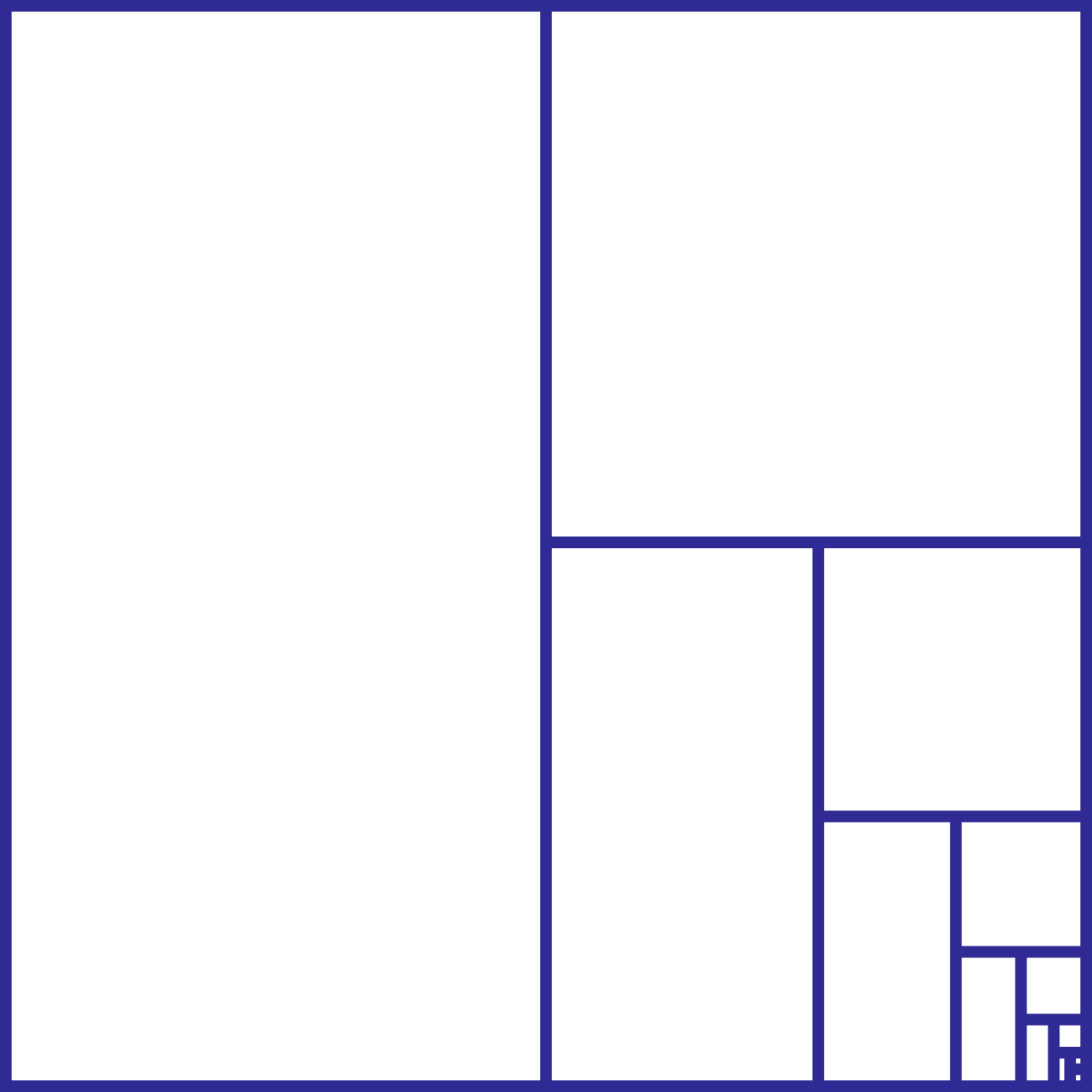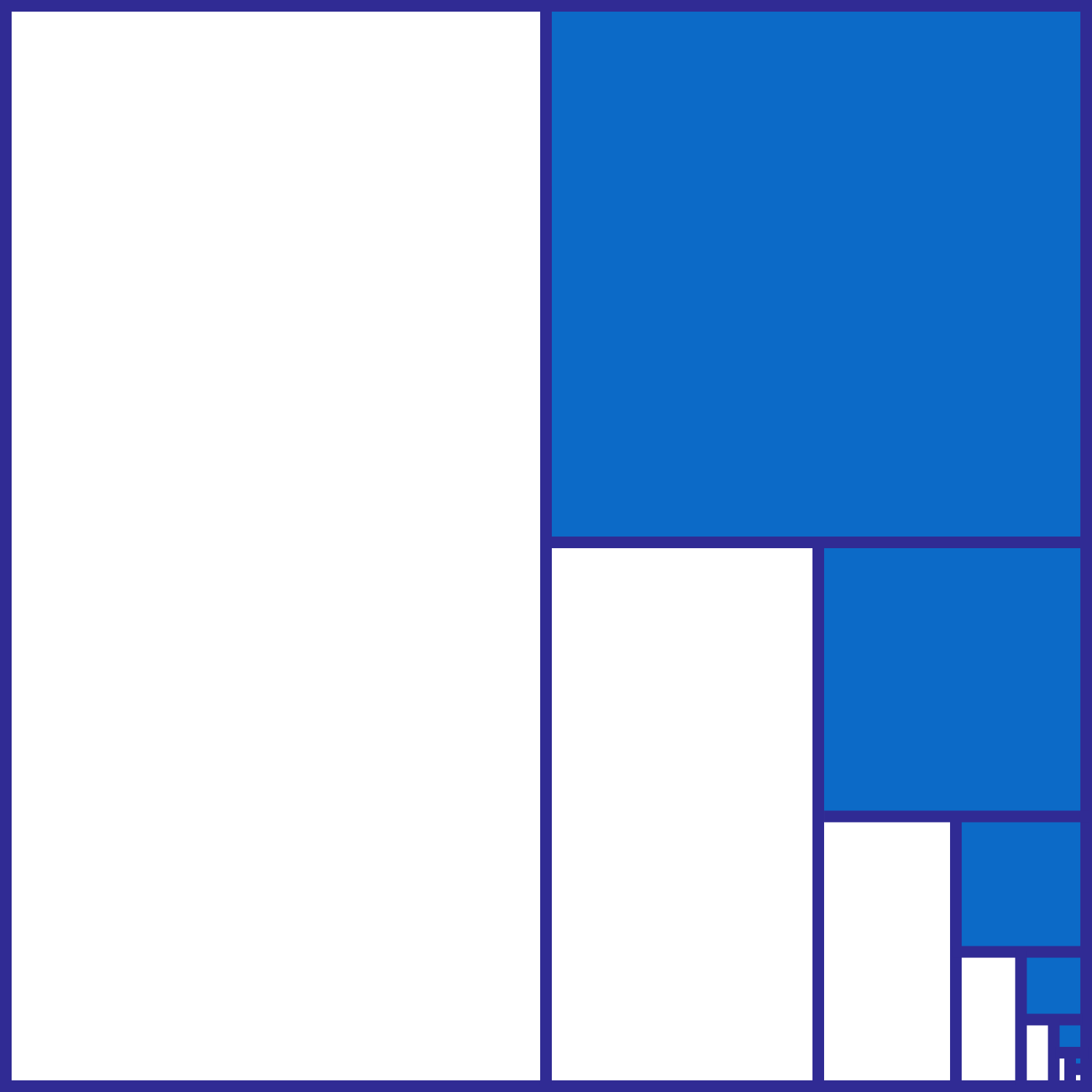# Half of a Series

Algebra Level 1It is well known that
$\frac{1}{2} + \frac{1}{4} + \frac{1}{8} + \frac{1}{16} + \cdots = 1.$ Now, the figure on the right shows a square successively divided into half.If we color the successively smaller squares blue, as shown on the left, what fraction of the largest square is shaded blue?

×